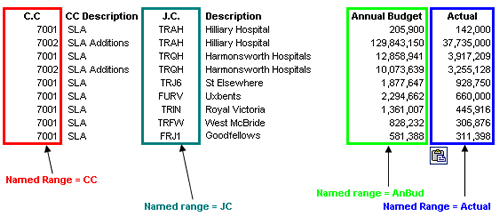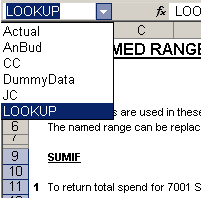Named ranges are useful in Excel as their use can make formulas much more understandable. You can name: areas of the spreadsheet, such as your data columns and rows or individual cells that may act as constants, for example the VAT value

I’ve used the following named ranges in the SUMIF example below:As usual with Excel, there are a number of ways to do the same thing. To name a range you can highlight the area and then either:

1. Insert>Name>Define and then choose an appropriate title or 2. Go to the name box in the top left hand corner of the formula bar and type a name in the cell

To confirm the region that the named range refers to, use the drop down Name Box and select the range of interest.The SUMIF function is in my list of all time useful functions, the excel help is very good, but here is my tutorial with real world finance examples. The SUMIF function adds the cells specified by a given criteria.

The formula syntax is:

`SUMIF(range,criteria,sum\_range)`

where “Range” is the range of cells you want evaluated. “Criteria” is the criteria in the form of a number, expression, or text that defines which cells will be added. “Sum_range” are the actual cells to sum.

Here are some uses of the SUMIF function, utilising the data sown above.

1. To return total spend for 7001 SLA’s: `=SUMIF(CC,7001,Actual)` where CC (Cost Centre) = 7001 return the sum of Actuals

2. To return the total annual budget for Hilliary Hospital SLA and Additions: `=SUMIF(JC,TRAH,AnBud)` where JC (Job Code) = TRAH return the sum of AnBud